Updating search results...

# 7 Results

View
Selected filters:
• UT.MATH.III.A.APR.2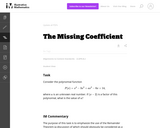Unrestricted Use
CC BY
Rating
0.0 stars

The purpose of this task is to emphasize the use of the Remainder Theorem (a discussion of which should obviously be considered as a prerequisite for the task) as a method for determining structure in polynomial in equations, and in this particular instance, as a replacement for division of polynomials.

Subject:
Mathematics
Secondary Mathematics
Material Type:
Activity/Lab
Provider:
Illustrative Mathematics
Provider Set:
Illustrative Mathematics
Author:
Illustrative Mathematics
05/01/2012Conditional Remix & Share Permitted
CC BY-NC
Rating
0.0 stars

This lesson plan is a unit on solving and building polynomials, modeling polynomials, and using transformations to graph functions.

Subject:
Secondary Mathematics
Material Type:
Unit of Study
Author:
Tyrani Bevell
10/14/2021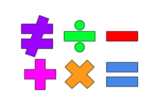Unrestricted Use
Public Domain
Rating
0.0 stars

This lesson is for a math classroom, but can be adapted to fit any grade, subject, or content.&nbsp;&nbsp;In this lesson, students will use an iPad and its features: Keynote, Pages, Garageband, Numbers, Presentation, and iMovie.&nbsp; Students will use 3 of those features/programs to create a video lesson consisting of several examples from a topic of their choice.&nbsp; This project is in place of a term final, so their chosen topic should be from their current term.Image citation: The image is one I created.&nbsp;&nbsp;

Subject:
Secondary Mathematics
Material Type:
Assessment
Author:
Tyrani Bevell
02/26/2022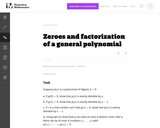Unrestricted Use
CC BY
Rating
0.0 stars

This task looks at zeroes and factorization of a general polynomial. It is related to a very deep theorem in mathematics, the Fundamental Theorem of Algebra, which says that a polynomial of degree d always has exactly d roots, provided complex numbers are allowed as roots and provided roots are counted with the proper "multiplicity.''

Subject:
Mathematics
Secondary Mathematics
Material Type:
Activity/Lab
Provider:
Illustrative Mathematics
Provider Set:
Illustrative Mathematics
Author:
Illustrative Mathematics
08/17/2012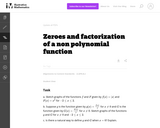Unrestricted Use
CC BY
Rating
0.0 stars

The intention of this task is to provide extra depth to the standard A-APR.2 it is principally designed for instructional purposes only. The students may use graphing technology: the focus, however, should be on what happens to the function g when x=0 and the calculator may or may not be of help here (depending on how sophisticated it is!).

Subject:
Mathematics
Secondary Mathematics
Material Type:
Activity/Lab
Provider:
Illustrative Mathematics
Provider Set:
Illustrative Mathematics
Author:
Illustrative Mathematics
08/21/2012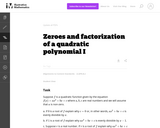Unrestricted Use
CC BY
Rating
0.0 stars

For a polynomial function p, a real number r is a root of p if and only if p(x) is evenly divisible by x_r. This fact leads to one of the important properties of polynomial functions: a polynomial of degree d can have at most d roots. This is the first of a sequence of problems aiming at showing this fact.

Subject:
Mathematics
Secondary Mathematics
Material Type:
Activity/Lab
Provider:
Illustrative Mathematics
Provider Set:
Illustrative Mathematics
Author:
Illustrative Mathematics
08/17/2012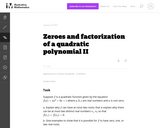Unrestricted Use
CC BY
Rating
0.0 stars

This task continues ``Zeroes and factorization of a quadratic polynomial I.'' The argument here generalizes, as shown in ``Zeroes and factorization of a general polynomial'' to show that a polynomial of degree d can have at most d roots. This task is intended for instructional purposes to help students see more clearly the link between factorization of polynomials and zeroes of polynomial functions.

Subject:
Mathematics
Secondary Mathematics
Material Type:
Activity/Lab
Provider:
Illustrative Mathematics
Provider Set:
Illustrative Mathematics
Author:
Illustrative Mathematics